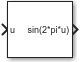# Sine, Cosine

Implement fixed-point sine or cosine wave using lookup table approach that exploits quarter wave symmetry

• Library:

••## Description

The Sine and Cosine block implements a sine and/or cosine wave in fixed point using a lookup table method that exploits quarter wave symmetry. The block can output the following functions of the input signal, depending upon what you select for the Output formula parameter:

• sin(2πu)

• cos(2πu)

• exp(ju)

• sin(2πu) and cos(2πu)

You define the number of lookup table points in the Number of data points for lookup table parameter. The block implementation is most efficient when you specify the lookup table data points to be `(2^n)+1`, where n is an integer.

Use the Output word length parameter to specify the word length of the fixed-point output data type. The fraction length of the output is the output word length minus 2.

## Ports

### Input

expand all

Input signal, u, specified as a real-valued scalar, vector, or matrix.

Tip

To obtain meaningful block output, the block input values should fall within the range [0, 1). For input values that fall outside this range, the values are cast to an unsigned data type, where overflows wrap. For these out-of-range inputs, the block output might not be meaningful.

Data Types: `single` | `double` | `int8` | `int16` | `int32` | `int64` | `uint8` | `uint16` | `uint32` | `uint64` | `Boolean` | `fixed point`

### Output

expand all

Fixed-point sine wave, implemented using a lookup table approach.

#### Dependencies

This port is enabled when the Output formula is set to `sin(2*pi*u)` or `sin(2*pi*u) and cos(2*pi*u)`.

Data Types: `fixed point`

Fixed-point cosine wave, implemented using a lookup table approach.

#### Dependencies

This port is enabled when the Output formula is set to `cos(2*pi*u)` or `sin(2*pi*u) and cos(2*pi*u)`.

Data Types: `fixed point`

`exp(j*2*pi*u)`, implemented using a lookup table approach.

#### Dependencies

This port is enabled when the Output formula is set to `exp(j*2*pi*u)`.

Data Types: `fixed point`

## Parameters

expand all

#### Programmatic Use

 Block Parameter: `Formula` Values: ```'sin(2*pi*u)' | 'cos(2*pi*u)' | 'exp(j*2*pi*u)' | 'sin(2*pi*u) and cos(2*pi*u)'```

The implementation is most efficient when you specify the lookup table data points to be `(2^n)+1`, where n is an integer. To be compatible with the Output word length parameter, the Number of data points for lookup table must be less than or equal to (2^(Output word length-2)+1).

#### Programmatic Use

 Block Parameter: `NumDataPoints` Type: scalar Value: `integer >= 2` Default: `'(2^5)+1'`

The fraction length of the output is the output word length minus 2. To be compatible with the Number of data points for lookup table parameter, (2^(Output word length - 2) +1) must be greater than or equal to Number of data points for lookup table.

Note

The block uses double-precision floating-point values to construct lookup tables. Therefore, the maximum amount of precision you can achieve in your output is 53 bits. Setting the word length to values greater than 53 bits does not improve the precision of your output.

#### Programmatic Use

 Block Parameter: `OutputWordLength` Type: scalar Value: `integer from 2 to 53` Default: `'16'`

Select `Speed` for faster calculations. If you do, a loss of accuracy might occur, usually up to 2 bits.

#### Programmatic Use

 Block Parameter: `InternalRulePriority` Values: `'Speed' | 'Precision'` Default: `'Speed'`

## Block Characteristics

 Data Types `Boolean` | `double` | `fixed point` | `integer` | `single` Direct Feedthrough `no` Multidimensional Signals `no` Variable-Size Signals `no` Zero-Crossing Detection `no`

## Version History

Introduced before R2006a